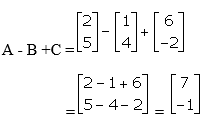# Selina Solutions Concise Maths Class 10 Chapter 9 Matrices Exercise 9(A)

The important topics which are explained under this exercise are equality of matrices, Addition, subtraction of matrices, additive identity, additive inverse and solving matrix equations. The Selina Solutions for Class 10 Maths is a one-stop solution to all doubts and clarifications in solving problems of this chapter and others. All the solutions are as per the latest ICSE examination patterns. The solutions of this exercise are available in the Concise Selina Solutions for Class 10 Maths Chapter 9 Matrices Exercise 9(A), PDF provided in the links given below.

## Selina Solutions Concise Maths Class 10 Chapter 9 Matrices Exercise 9(A) Download PDF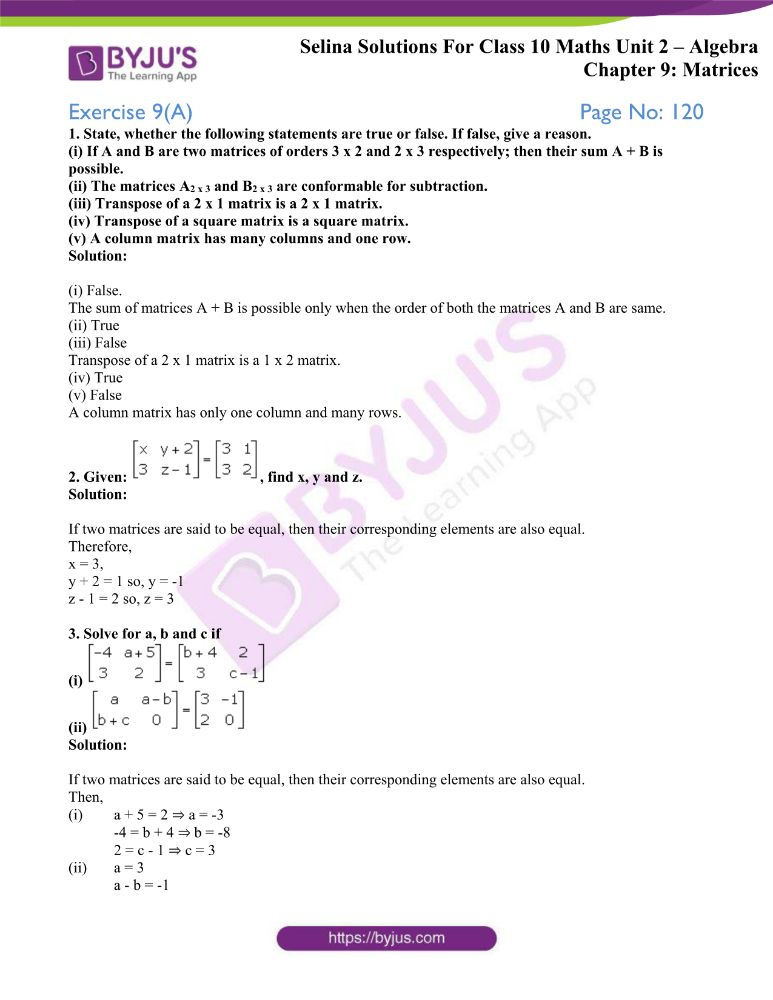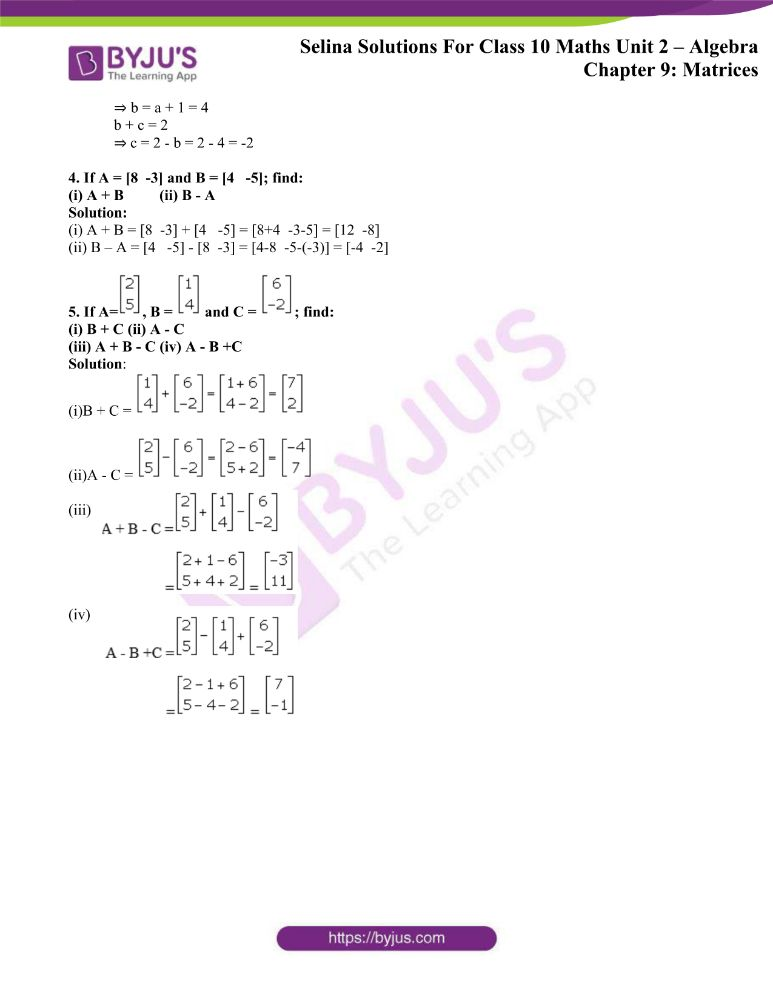### Access other exercises of Selina Solutions Concise Maths Class 10 Chapter 9 Matrices

Exercise 9(B) Solutions

Exercise 9(C) Solutions

Exercise 9(D) Solutions

### Access Selina Solutions Concise Maths Class 10 Chapter 9 Matrices Exercise 9(A)

1. State, whether the following statements are true or false. If false, give a reason.

(i) If A and B are two matrices of orders 3 x 2 and 2 x 3 respectively; then their sum A + B is possible.

(ii) The matrices A2 x 3 and B2 x 3 are conformable for subtraction.

(iii) Transpose of a 2 x 1 matrix is a 2 x 1 matrix.

(iv) Transpose of a square matrix is a square matrix.

(v) A column matrix has many columns and one row.

Solution:

(i) False.

The sum of matrices A + B is possible only when the order of both the matrices A and B are same.

(ii) True

(iii) False

Transpose of a 2 x 1 matrix is a 1 x 2 matrix.

(iv) True

(v) False

A column matrix has only one column and many rows.

2. Given: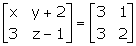, find x, y and z.

Solution:

If two matrices are said to be equal, then their corresponding elements are also equal.

Therefore,

x = 3,

y + 2 = 1 so, y = -1

z – 1 = 2 so, z = 3

3. Solve for a, b and c if

(i)(ii)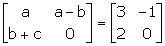Solution:

If two matrices are said to be equal, then their corresponding elements are also equal.

Then,

(i) a + 5 = 2 ⇒ a = -3

-4 = b + 4 ⇒ b = -8

2 = c – 1 ⇒ c = 3

(ii) a = 3

a – b = -1

⇒ b = a + 1 = 4

b + c = 2

⇒ c = 2 – b = 2 – 4 = -2

4. If A = [8 -3] and B = [4 -5]; find:

(i) A + B (ii) B – A

Solution:

(i) A + B = [8 -3] + [4 -5] = [8+4 -3-5] = [12 -8]

(ii) B – A = [4 -5] – [8 -3] = [4-8 -5-(-3)] = [-4 -2]

5. If A=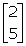, B =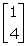and C =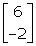; find:

(i) B + C (ii) A – C

(iii) A + B – C (iv) A – B +C

Solution:

(i)B + C =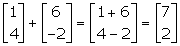(ii)A – C =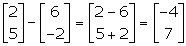(iii)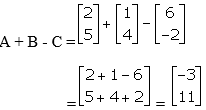(iv)